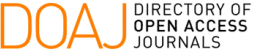### Asymptotic Behaviour of a Viscoelastic Transmission Problem with a Tip Load

J. E. M. Rivera, M. S. Carneiro, M. A. A. Fernandes

#### Abstract

We consider a transmission problem for a string composed by two components: one of them is a viscoelastic material (with viscoelasticity of memory type), and the other is an elastic material (without dissipation effective over this component). Additionally, we consider that in one end is attached a tip load. The main result is that the model is exponentially stable if and only if the memory effect is effective over the string. When there is no memory effect, then there is a lack of exponential stability, but the tip load produces a polynomial rate of decay. That is, the tip load is not strong enough to stabilize exponentially the system, but produces a polynomial rate of decay.

#### Keywords

Transmission problem; memory effect; lack of exponential stability.

PDF

#### References

K. Andrews and M. Shillor, “Vibrations of a beam with a damping tip body,” Mathematical and Computer Modelling, vol. 35, pp. 1033–1042, 2002.

N. Zietsman, L Van Rensburg and A. Van der Merwe, “A timoshenko beam with tip body and boundary damping,” Wave Motion, vol. 39, pp. 199–211, 2004.

E. Feireisl and G. O’Dowd, “Stabilisation d’un système hybride par un feedback non linéaire, non monotone,” Comptes Rendus de l’Académie des Sciences, vol. 326, pp. 323–327, 1998.

C. M. Dafermos, “On abstract volterra equation with applications to linear viscoleasticity,” Differential and Integral Equations, vol. 7, pp. 554–569, 1970.

C. M. Dafermos, “Asymptotic stability in viscoleasticity,” Arch. Rat. Mech. Anal., vol. 37, pp. 297–308, 1970.

M. Fabrizio and A. Morro, Asymptotic Stability in Viscoleasticity, vol. 12 of SIAM Studies in Applied Mathematics. Philadelphia: Society for Industrial and Applied Mathematics, 1992.

J. Prüss, “On the spectrum of c0-semigroups,” Trans. AMS, vol. 284, pp. 847– 857, 1984.

M. Reed and B. Simon, Methods of Modern Mathematical Physics, vol. 04. California: Academic Press Inc., 1978.

T. Kato, Perturbation Theory for Linear Operators. New York: Springer- Verlag, 1980.

K. J. Engel and R. Nagel, One-parameter Semigroups for Linear Evolution Equations, vol. 194 of Graduate Texts in Mathematics. New York: Springer- Verlag, 2000.

A. Borichev and Y. Tomilov, “Optimal polynomial decay of functions and op- erator semigroups,” Math. Ann., vol. 347, pp. 455–478, 2009.

DOI: https://doi.org/10.5540/tcam.2023.024.02.00357

### Refbacks

• There are currently no refbacks.

Trends in Computational and Applied Mathematics

A publication of the Brazilian Society of Applied and Computational Mathematics (SBMAC)

Indexed in: